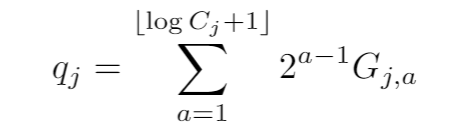# How to use cvx expression

Hi everyone,

I am new to using cvx expressions and would like to know if what I have implemented is correct. I wish to express the following summation:My code:

J = 5; C = randi([100 8e2],5,1);
variable G(5,amax+1) binary;
expression ib4(5);
for j = 1:J
temp1(j) = floor(log2(C(j)));
for a = 1:temp1(j)+1
ib4(j) = ib4(j) + 2^(a-1) * G(j,a);
end
end

Thanks!

That looks o.k. other than I don’'t know why you don’t have the `+1` in `floor(log2(C(j))+1);` But that’s not a CVX matter.

All elements of CVX expression holders are initialized to zero, which is a behavior your code relies on.

Try inputting numerical values of `G` instead of declaring it a variable, using `b4=zeros(5,1) instead of expression, and verify it evaluates to what you want.

Thank you @Mark_L_Stone for the suggestion and confirmation. I declared G as all ones and ib4 as all zeros and got correct results.